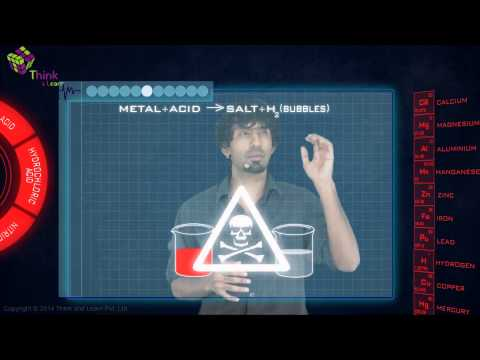# Extraction of Crude Metal From Concentrated Ore

## What is of Crude Metal?

Metal extraction from a concentrated solution The refining of metals from the compounds in which they are found to their original condition is known as ore. After converting the concentrated ore to an oxide, the metal is extracted by reducing the oxide to metal with an appropriate reducing agent. Extraction of metals occur over three major stages.

1. Concentration of Ore
2. Isolation of metal from the concentrated Ore
3. Purification of the metal
Isolation of metal from the concentrated Ore involves the refining of metals from the compounds it is found with to its original state. The concentrated ore is first converted to an oxide after which metal extraction is done by reducing it from an oxide to metal using a suitable reducing agent.

## Conversion to Oxide

In this process, metal refining is done by heating the ore in a limited supply or absence of air. This is done with the aim of bringing about thermal decomposition and remove volatile compounds from the ore leaving behind a metal oxide.

Fe2O3.xH2O(s)  $$\require{AMScd} \begin{CD} @>▲>> \end{CD}$$ Fe2O3 (s) + xH2O(g)

CaCO3.MgCO3(s)   $$\require{AMScd} \begin{CD} @>▲>> \end{CD}$$ CaO(s) + MgO(s) + 2CO2(g)

## Roasting

Metal extraction here is done by heating the ore with a regular supply of hot air in the furnace. This is done to encourage solid-gas reaction between the ore and the air. Sulphide ores of elements such as Zinc, Lead and Copper are treated using this method.

The sulphur in the ore, at elevated temperature, reacts with the oxygen to create Sulphur Dioxide and escapes out of the ore into the air.

$$~~~~~~~~~~~$$2ZnS      +    3O2         $$\require{AMScd} \begin{CD} @>▲>> \end{CD}$$     2ZnO + 2SO2
$$~~~~~~~~~~~$$2PbS      +     3O2    $$\require{AMScd} \begin{CD} @>▲>> \end{CD}$$     2PbO + 2SO2
$$~~~~~~~~~~~$$2Cu2S    +     3O2    $$\require{AMScd} \begin{CD} @>▲>> \end{CD}$$ 2Cu2O + 2SO2

## Reduction of Oxide to Metal

Reduction of metal oxide means removal of oxygen to refine metal to its free state. This is done with the help of reducing agents such as Carbon (C) and Carbon Monoxide (CO). Carbon is added to the metal oxide obtained from the ore and heated.

The carbon under the right condition reacts with metal oxide and takes the oxygen with it thereby reducing the metal and leaving as carbon monoxide gas. If CO is used as reducing agent then the gas produced will be Carbon dioxide.

$$~~~~~~~~~~~$$MxOy    +      yC        $$\require{AMScd} \begin{CD} @>▲>> \end{CD}$$ xM + y CO
$$~~~~~~~~~~~$$MxOy    +      yCO     $$\require{AMScd} \begin{CD} @>▲>> \end{CD}$$ xM + y CO2

## Recommended VideosWe use so many kinds of metals in our daily life and the refining of metals and its eventual extraction and usage affects all of us directly. Metallurgy is a vast treasure-trove of essential knowledge. Come explore it with expert tutors at BYJU’S.

Quiz on Extraction Of Crude Metal From Concentrated Ore